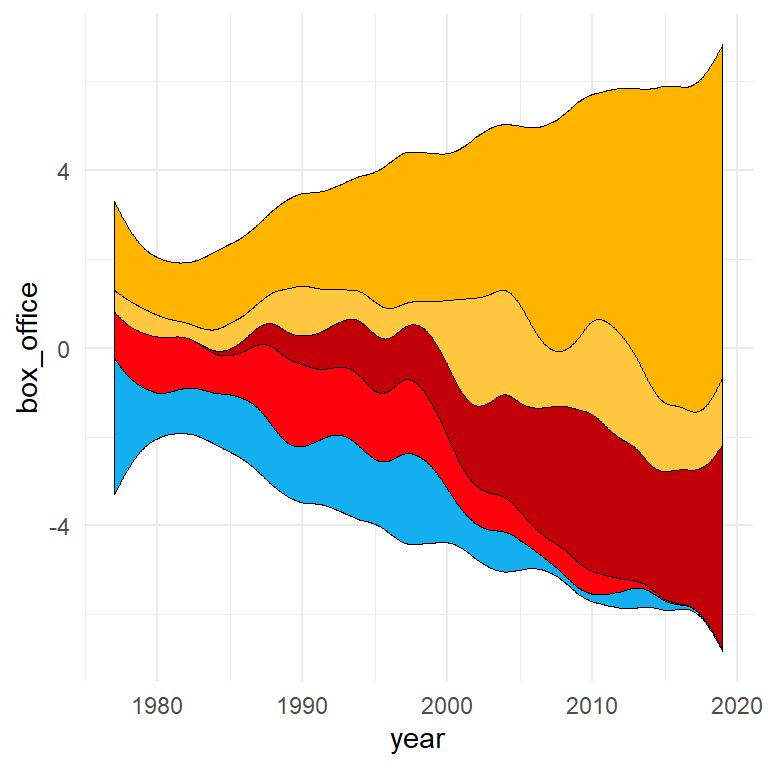# Streamgraph in ggplot2

David Sjoberg

## Sample data

Consider the `blockbusters` data set from the `ggstream` package for this guide.

``````# install.packages("remotes")
# remotes::install_github("davidsjoberg/ggstream")
library(ggstream)

blockbusters``````

## Stream plots in ggplot2 with `geom_stream`

The `geom_stream` function of the `ggstrean` package allows creating streamplots in ggplot2. Pass your data and use the function to create a basic stream graph.

``````# install.packages("remotes")
# remotes::install_github("davidsjoberg/ggstream")
library(ggstream)
# install.packages("ggplot2")
library(ggplot2)

ggplot(blockbusters, aes(x = year, y = box_office, fill = genre)) +
geom_stream()``````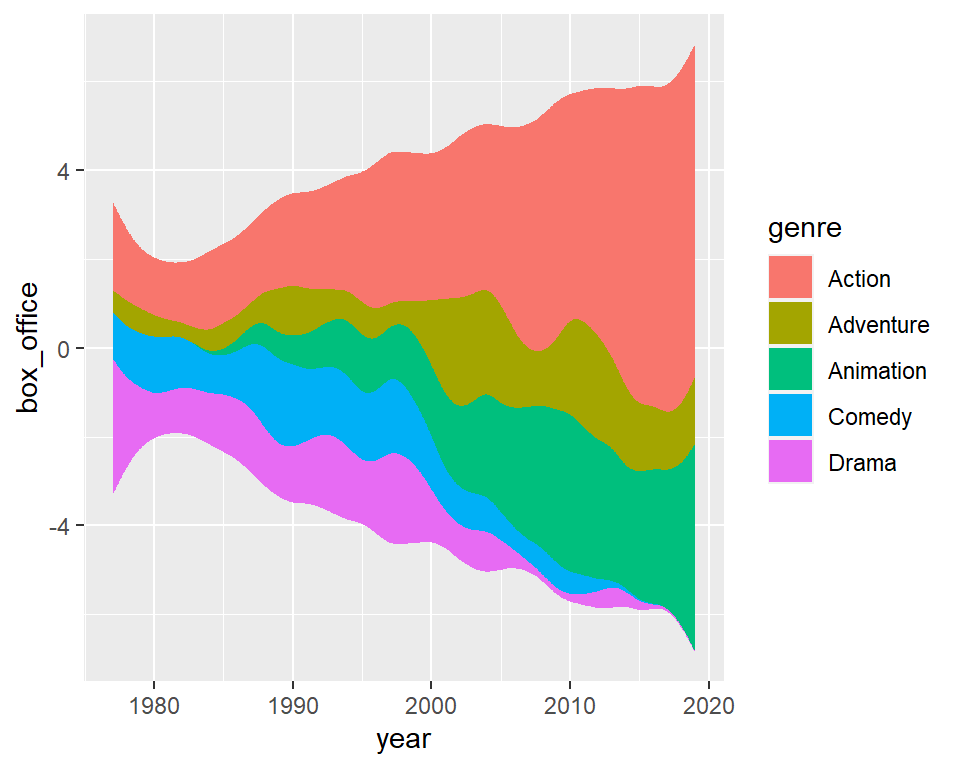Note that the package contains an additional function named `geom_stream_label` that can be used to add labels to each area of the streamgraph.

``````# install.packages("remotes")
# remotes::install_github("davidsjoberg/ggstream")
library(ggstream)
# install.packages("ggplot2")
library(ggplot2)

ggplot(blockbusters, aes(x = year, y = box_office, fill = genre)) +
geom_stream() +
geom_stream_label(aes(label = genre))``````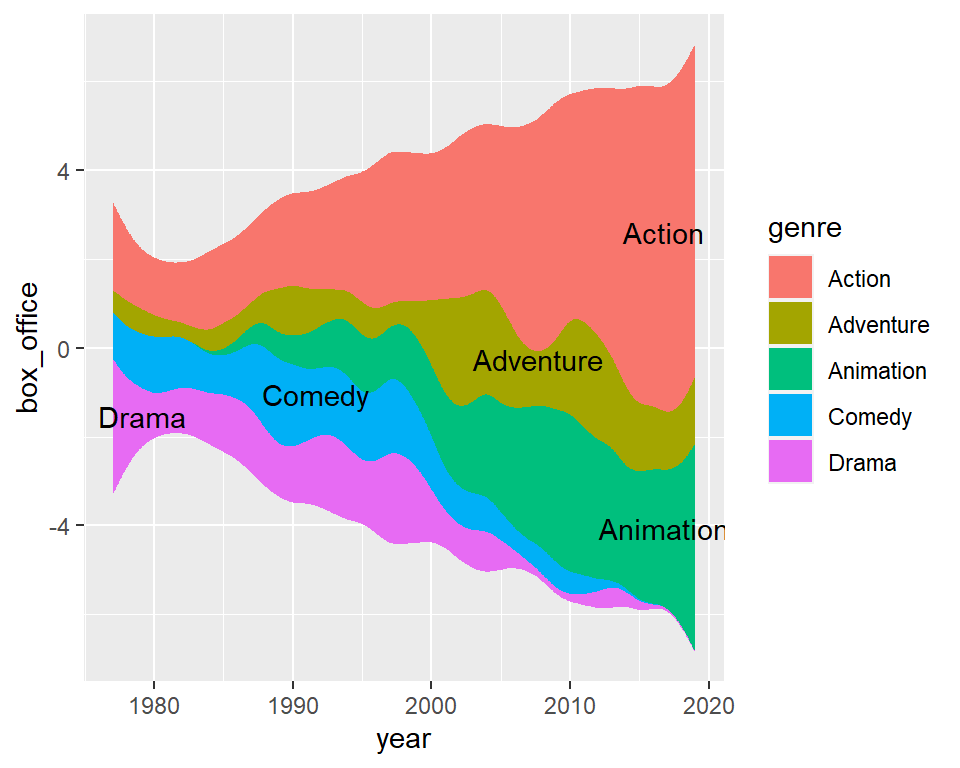## Stream graphs types

By default, the `geom_stream` function uses the `"mirror"` type, which stacks the streams symmetrically around the X-axis. The other alternatives are `"ridge"`, which stacks from the X-axis and `"proportional"`, which makes the streams sum up to 1.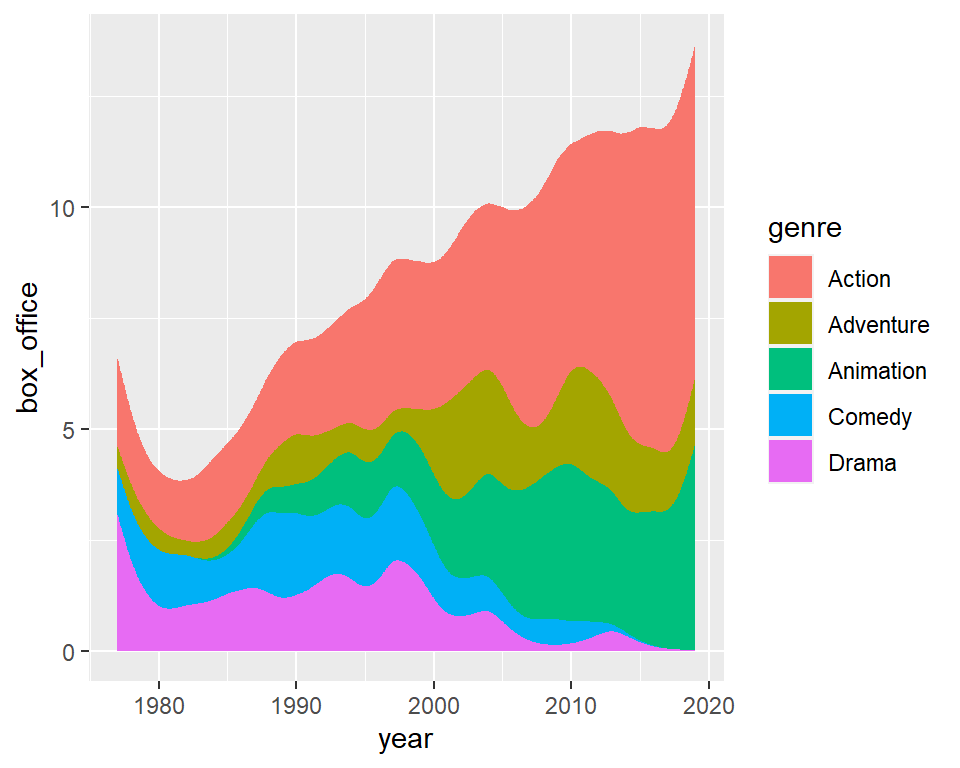Ridge

``````# install.packages("remotes")
# remotes::install_github("davidsjoberg/ggstream")
library(ggstream)
# install.packages("ggplot2")
library(ggplot2)

ggplot(blockbusters, aes(x = year, y = box_office, fill = genre)) +
geom_stream(type = "ridge")``````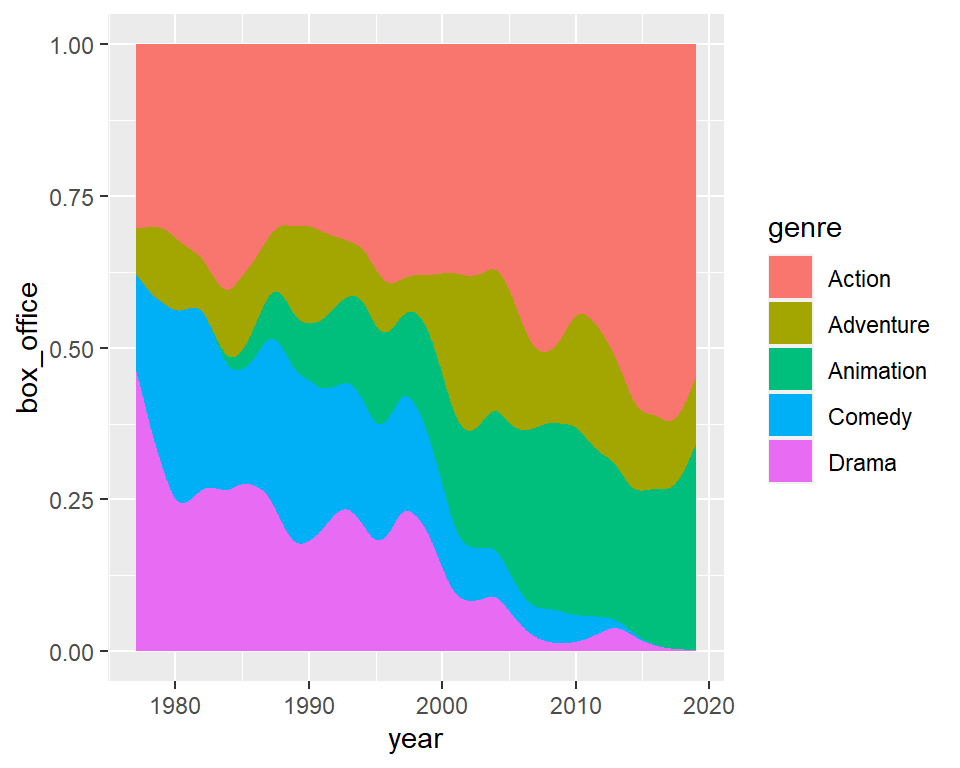Proportional

``````# install.packages("remotes")
# remotes::install_github("davidsjoberg/ggstream")
library(ggstream)
# install.packages("ggplot2")
library(ggplot2)

ggplot(blockbusters, aes(x = year, y = box_office, fill = genre)) +
geom_stream(type = "proportional")``````

## Color customization

Change the fill colors

Is is possible to change the fill colors of the streamgraph with `scale_fill_manual` or an equivalent function.

``````# install.packages("remotes")
# remotes::install_github("davidsjoberg/ggstream")
library(ggstream)
# install.packages("ggplot2")
library(ggplot2)

cols <- c("#FFB400", "#FFC740", "#C20008", "#FF020D", "#13AFEF")

ggplot(blockbusters, aes(x = year, y = box_office, fill = genre)) +
geom_stream() +
scale_fill_manual(values = cols)``````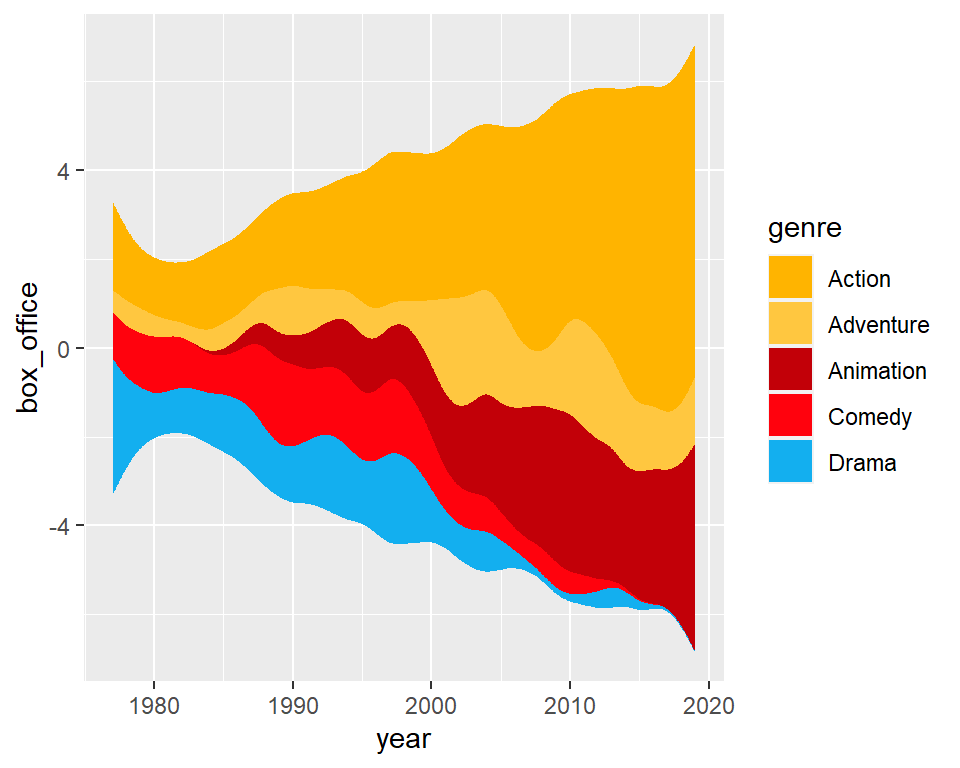Change the color of the borders

By default the areas have no border but you can add a border color with the `color` argument of the `geom_stream` function and modify its width with `lwd`.

``````# install.packages("remotes")
# remotes::install_github("davidsjoberg/ggstream")
library(ggstream)
# install.packages("ggplot2")
library(ggplot2)

cols <- c("#FFB400", "#FFC740", "#C20008", "#FF020D", "#13AFEF")

ggplot(blockbusters, aes(x = year, y = box_office, fill = genre)) +
geom_stream(color = 1, lwd = 0.25) +
scale_fill_manual(values = cols)``````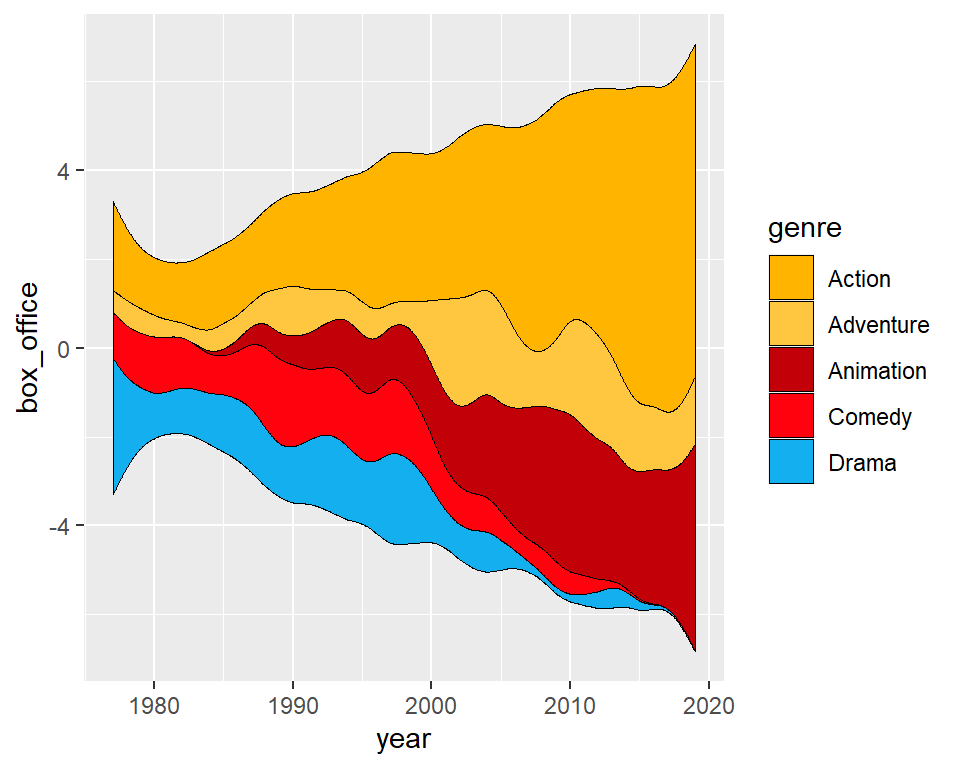Change the theme

Note that you can also change the theme to modify the appearance of the chart.

``````# install.packages("remotes")
# remotes::install_github("davidsjoberg/ggstream")
library(ggstream)
# install.packages("ggplot2")
library(ggplot2)

cols <- c("#FFB400", "#FFC740", "#C20008", "#FF020D", "#13AFEF")

ggplot(blockbusters, aes(x = year, y = box_office, fill = genre)) +
geom_stream(color = 1, lwd = 0.25) +
scale_fill_manual(values = cols) +
theme_minimal()``````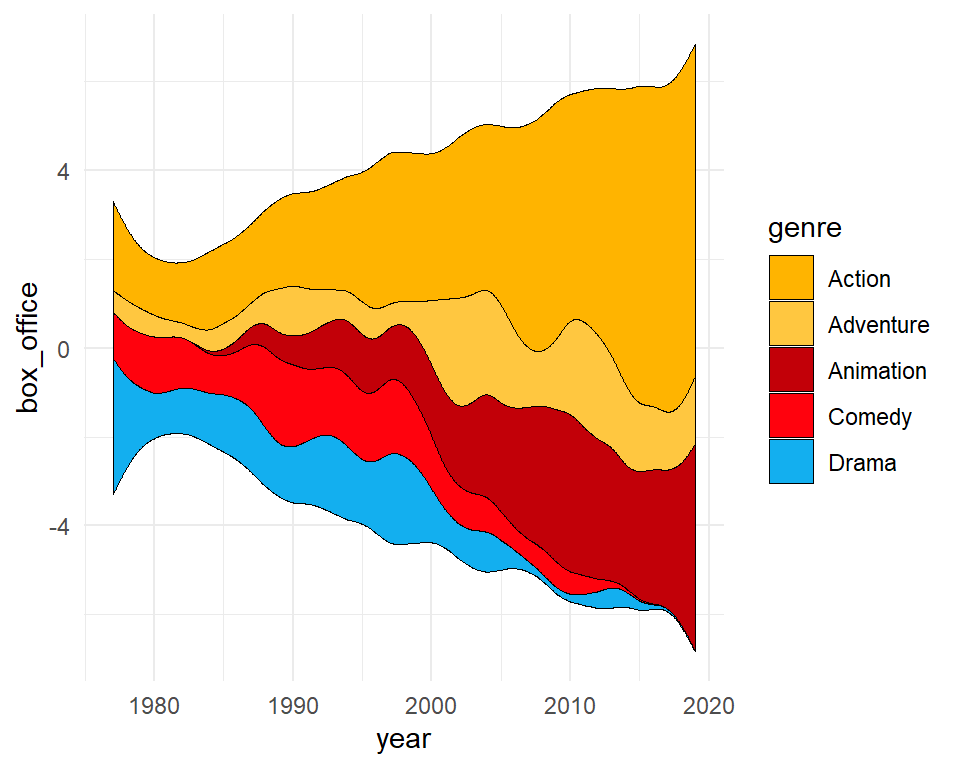In order to create a streamplot there are underlying non-parametric computations which need a bandwidth (defaults to 0.75) and a grid of values on the X-axis (defaults to 1000). You can change these default values with `bw` and `n_grid`.

Note that you can also use an extra range in the estimation with `extra_span`, that defaults to 0.01 (1%) and specify if the true range of the data should be used on the visualization or the estimation range with `true_range`.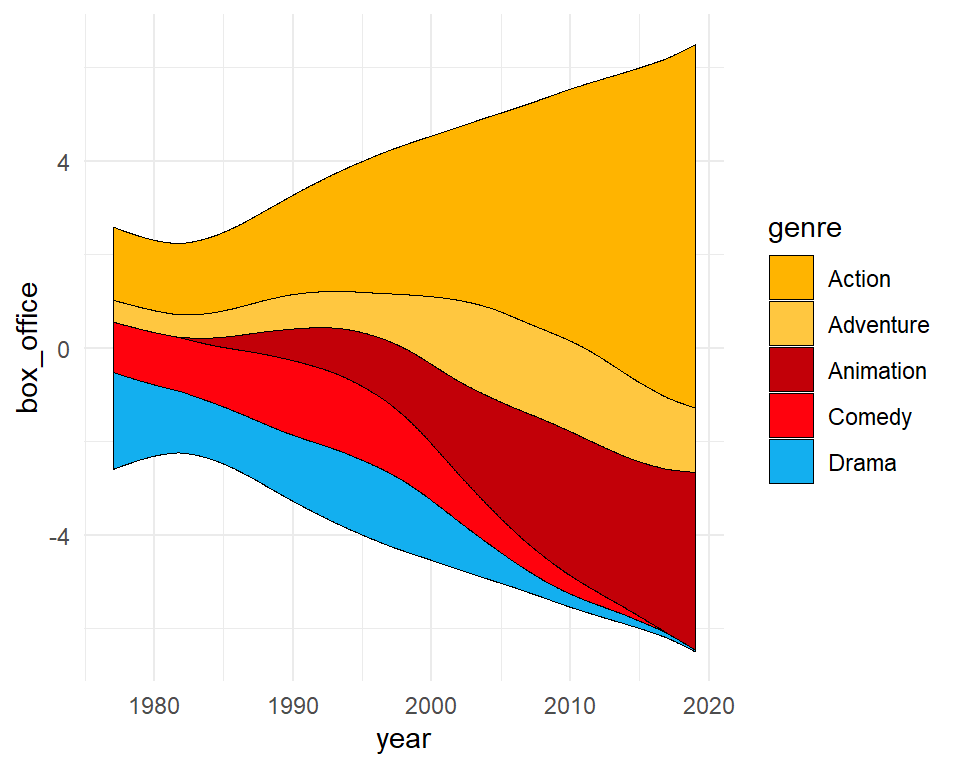Bandwidth (`bw`)

``````# install.packages("remotes")
# remotes::install_github("davidsjoberg/ggstream")
library(ggstream)
# install.packages("ggplot2")
library(ggplot2)

cols <- c("#FFB400", "#FFC740", "#C20008", "#FF020D", "#13AFEF")

ggplot(blockbusters, aes(x = year, y = box_office, fill = genre)) +
geom_stream(color = 1, lwd = 0.25,
bw = 1) +
scale_fill_manual(values = cols) +
theme_minimal()``````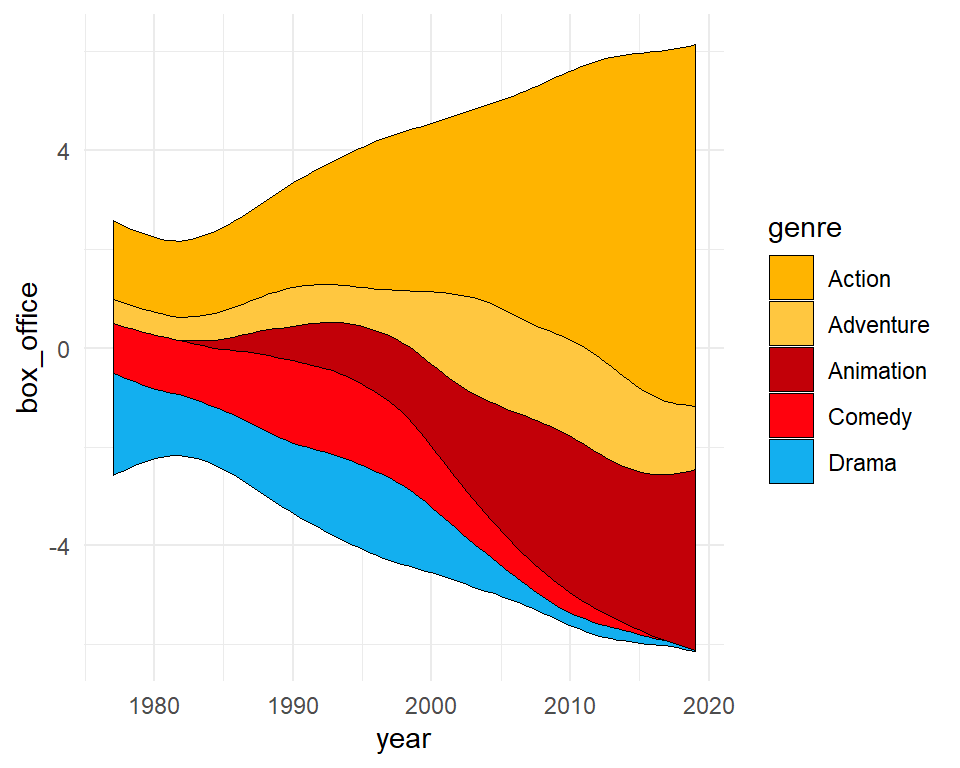Grid (`n_grid`)

``````# install.packages("remotes")
# remotes::install_github("davidsjoberg/ggstream")
library(ggstream)
# install.packages("ggplot2")
library(ggplot2)

cols <- c("#FFB400", "#FFC740", "#C20008", "#FF020D", "#13AFEF")

ggplot(blockbusters, aes(x = year, y = box_office, fill = genre)) +
geom_stream(color = 1, lwd = 0.25,
n_grid = 100) +
scale_fill_manual(values = cols) +
theme_minimal()``````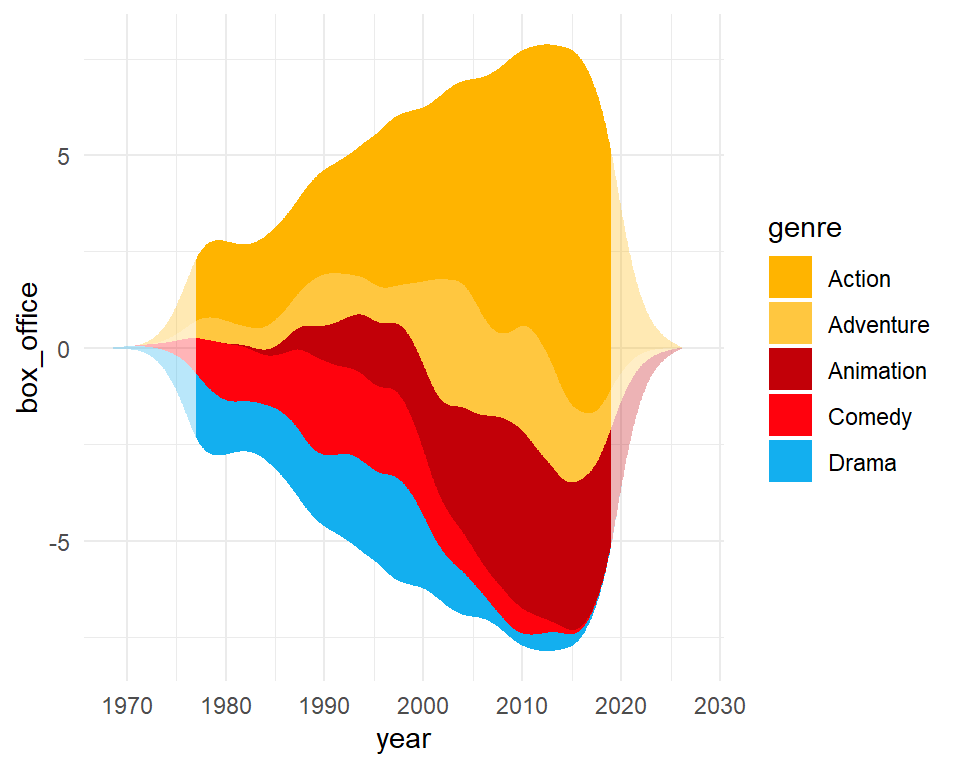Extra span and true range (`extra_span`, `true_range`)

``````# install.packages("remotes")
# remotes::install_github("davidsjoberg/ggstream")
library(ggstream)
# install.packages("ggplot2")
library(ggplot2)

cols <- c("#FFB400", "#FFC740", "#C20008", "#FF020D", "#13AFEF")

ggplot(blockbusters, aes(x = year, y = box_office, fill = genre)) +
geom_stream(extra_span = 0.2) +
geom_stream(extra_span = 0.2, true_range = "none",
alpha = 0.3) +
scale_fill_manual(values = cols) +
theme_minimal()``````

## Legend customization

As in other ggplot2 charts you can change the title, the key labels and change the position or remove the legend, as shown in the examples below.

Change the title

``````# install.packages("remotes")
# remotes::install_github("davidsjoberg/ggstream")
library(ggstream)
# install.packages("ggplot2")
library(ggplot2)

cols <- c("#FFB400", "#FFC740", "#C20008", "#FF020D", "#13AFEF")

ggplot(blockbusters, aes(x = year, y = box_office, fill = genre)) +
geom_stream(extra_span = 0.2) +
geom_stream(extra_span = 0.2, true_range = "none",
alpha = 0.3) +
scale_fill_manual(values = cols) +
theme_minimal() +
guides(fill = guide_legend(title = "Title"))``````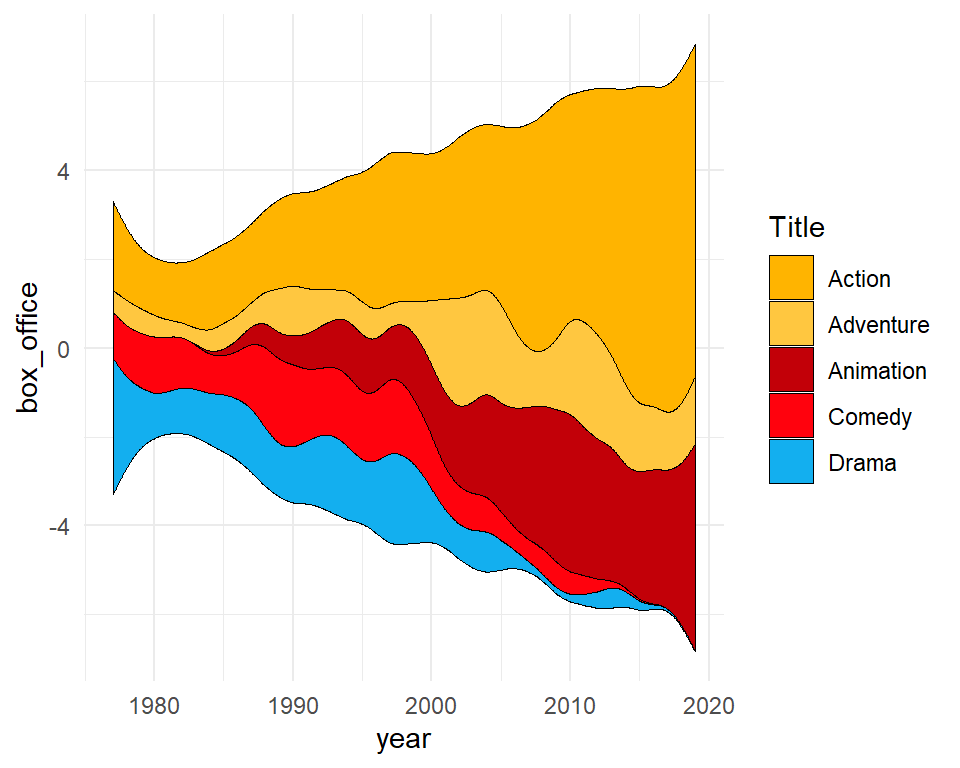Change the labels

``````# install.packages("remotes")
# remotes::install_github("davidsjoberg/ggstream")
library(ggstream)
# install.packages("ggplot2")
library(ggplot2)

cols <- c("#FFB400", "#FFC740", "#C20008", "#FF020D", "#13AFEF")

ggplot(blockbusters, aes(x = year, y = box_office, fill = genre)) +
geom_stream(color = 1, lwd = 0.25) +
scale_fill_manual(values = cols, labels = LETTERS[1:5]) +
theme_minimal()``````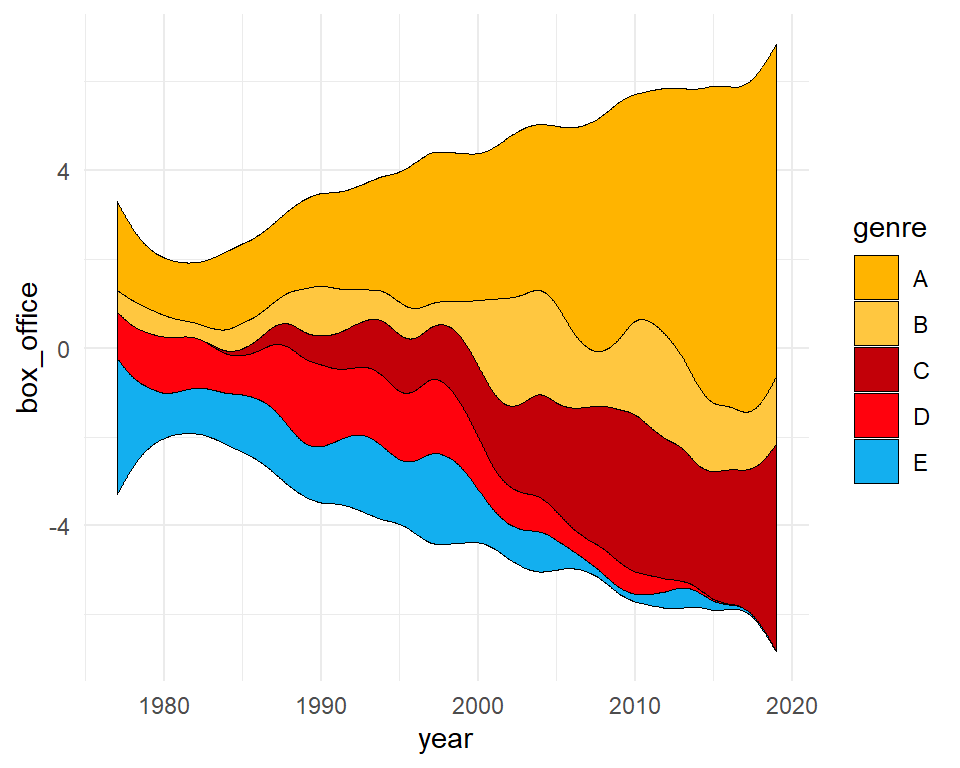Remove the legend

``````# install.packages("remotes")
# remotes::install_github("davidsjoberg/ggstream")
library(ggstream)
# install.packages("ggplot2")
library(ggplot2)

cols <- c("#FFB400", "#FFC740", "#C20008", "#FF020D", "#13AFEF")

ggplot(blockbusters, aes(x = year, y = box_office, fill = genre)) +
geom_stream(color = 1, lwd = 0.25) +
scale_fill_manual(values = cols) +
theme_minimal() +
theme(legend.position = "none")``````